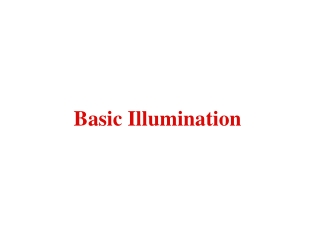DownloadDownload PresentationBasic Illumination

# Basic Illumination

Télécharger la présentation## Basic Illumination

- - - - - - - - - - - - - - - - - - - - - - - - - - - E N D - - - - - - - - - - - - - - - - - - - - - - - - - - -
##### Presentation Transcript

1. Basic Illumination

2. Depth Shading • Color or intensity determined solely by "depth" of polygon. • Darker colors or intensities at lower elevations. • Effective in modeling terrain or surface data • Avoids complex calculations of lighting dependent models • Simulates realism Depth Cueing • Reduce intensity of pixel as the distance from the observer increases • Simulates reduction in clarity as distances from the observer increases • Image fades in the distance • Often used in medical imaging Light Source Independent Models

3. What an object looks like depends on • Properties of the light source such as color, distance from object, direction from object, intensity of source • Surface characteristics of object such as color and reflectance properties • Location of the observer Light striking a surface of an object can be • Reflected (Diffuse reflection & Specular reflection) • Absorbed • Transmitted (Translucent or transparent) • Combination of all three Light Source Dependent Models

4. Lambert's Law - The intensity of light reflected from a surface is proportional to the cosine of the angle between the vector L to the light source and the normal vector N perpendicular to the surface. Diffuse Reflection using Lambert's Law The amount of reflected light is dependent on the position of the light source and the object but independent of the observer's position.

5. Let I = Illumination intensity Ip = Point light source intensity (white light) kd = Surface reflection coefficient (0<=kd<=1) ß = must be between 0 and 90 degrees A simple illumination model: I = Ipkd(cosß) Since cosß = (L•N)/(||L|| ||N||), then if L and N have unit length then we can use I = Ipkd (L•N) Simple Illumination Model

6. Ambient light is the illumination of an object caused by reflected light from other surfaces. To calculate this exactly would be very complicated. A simple model assumes ambient light is uniform in the environment. Let Ia = Ambient light intensity ka = Ambient light reflected Then we modify our previous illumination model to I = Iaka + Ipkd (L•N) Ambient Illumination

7. Thus far we have ignored the inverse square law: energy decays with the inverse square of the distance dL to the light source. Including this term we get I = Iaka + Ipkd (L•N)/dL2 However, due to our previous assumptions of a point light source and uniform ambient light, using the dL2 term gives too rapid of a decrease in illumination intensity to look realistic. The 1/dL2 term is usually replaced by fatt where fatt = MIN (1/(c1 + c2dL + c3dL2), 1) I = Iaka + Ipkd (N•L)*fatt Light-source Attenuation

8. •Light bounces off a glossy surface maintaining the color of the light source. •Visible when the angle of incidence of the light from the point light source is equal to the angle of reflection toward the observer. •For a non-perfect reflector, intensity of reflected light decreases rapidly as angle to observer increases beyond the angle of incidence. Specular Reflection N R L V = Observer Position N = Normal Vector L = Light Src Vector R = Reflected Vector V a ß ß

9. Ipfatt W(ß) cosna Ip = Point light source intensity fatt = Light-source Attenuation W(ø) = Fraction of specullarly reflected light (usually a constant, 0 < = ks) <= 1 n = Specular reflection exponent (perfect reflector n = ∞) cos a = R • V = (2N(N • L) – L) • V I = Iaka + Ipkd (N•L)*fatt + Specular Component Specular Component = Ipfatt ks(((2N(N • L) – L) • V)n This term represents the amount of the light source’s color that should be added! Phong's Highlighting Term

10. Given an RGB Color Space (A) Ambient Light = Ia (D) Diffuse Reflection = Ip (L • N)/(||L|| ||N||) fatt (S) Specular Reflection = Ip*fatt (R • V)n Given a White Light Source L = (255, 255, 255) Given a RGB point O = (R, G, B) Implementation Details Ir = Or(A*ka + D*kd) + Lr(S*ks) Ig = Og(A*ka + D*kd) + Lg(S*ks) Ib = Ob(A*ka + D*kd) + Lb(S*ks)